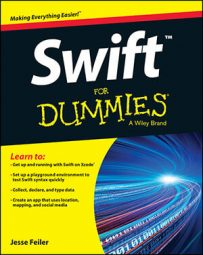##### Swift For DummiesConstants and variables have to be initialized before use in Swift. However, there’s more than one way to do this and get on with your code. If you’re not sure whether your approach will work, test out your initialization strategies in a playground. Here’s how:

1. Create a new playground with a single declaration, like this one:

`var x`
2. Try using your variable, x, in some way, like this:

`x = x + 2`

In this case, you’ll get an error.

3. To address the error, add an initializer to your declaration, like this:

`var x = 2`

This takes care of the problem.

Inside a class or structure, you use an init for each stored property. Here’s an example:

```struct myStruct {
var myStructVal: Double
init (fromConstant my100: Double) {
self.myStructVal = 100
}
init (fromParam myVal: Double) {
self.myStructVal = myVal
}
init () {
self.myStructVal = 1000;
}
}```

Here are the strategies:

• Initialize from a default value. Example:

```init () {
self.myStructVal = 1000;
}```
• Initialize with a constant ignoring any values passed in. This might be useful in testing. Example:

```init (fromConstant my100: Double) {
self.myStructVal = 100
}```
• Initialize with a parameter. You can use its value of perform a calculation with the parameter’s value. Example:

```init (fromParam myVal: Double) {
self.myStructVal = myVal
}```